# Chapter3.3prealg

22. Sep 2013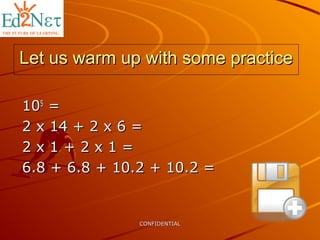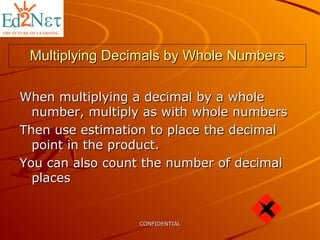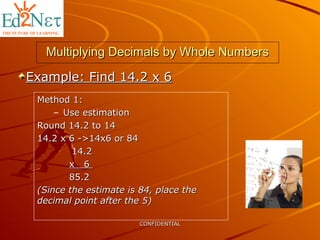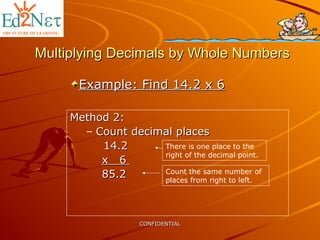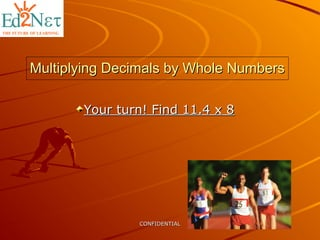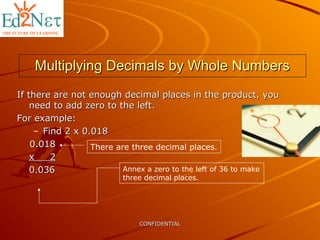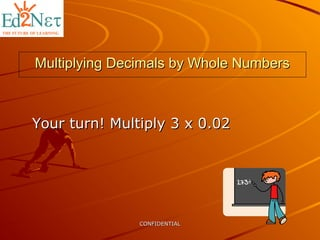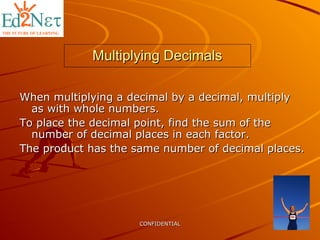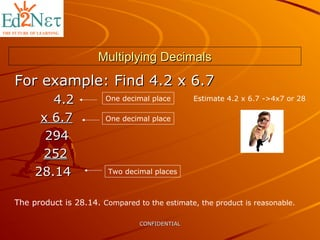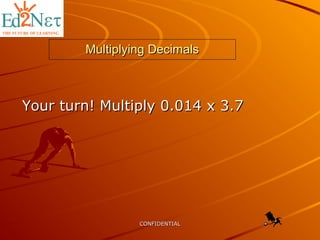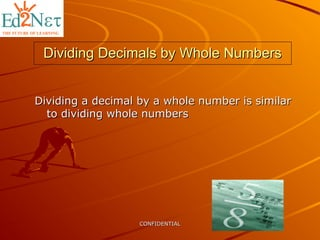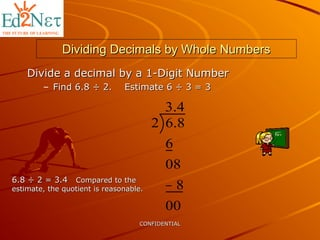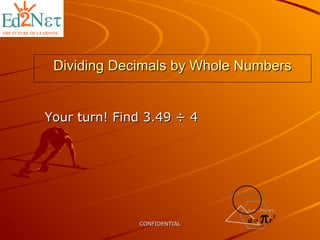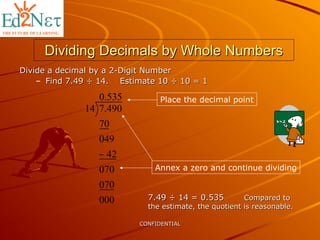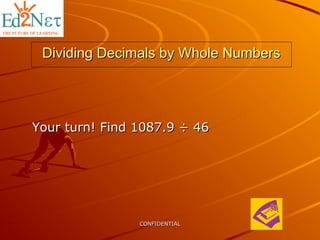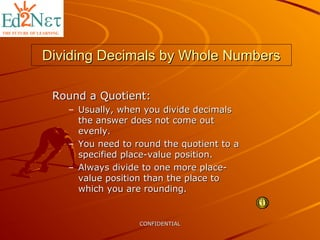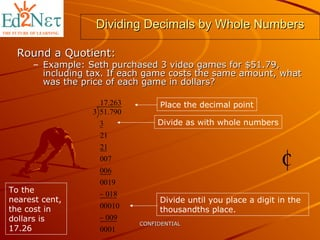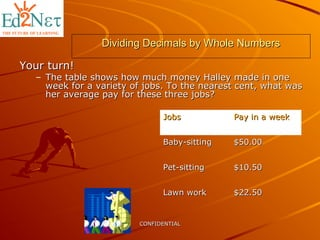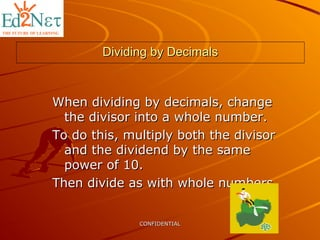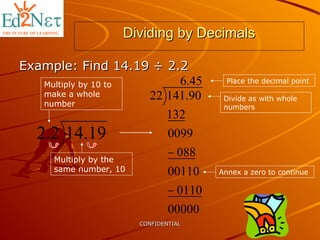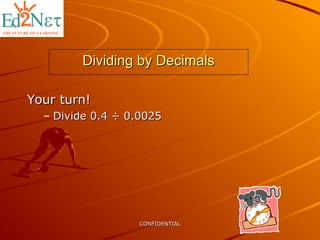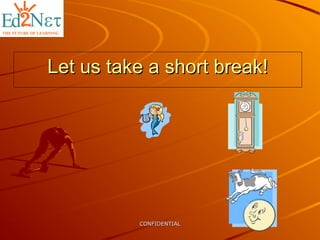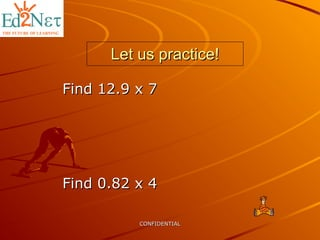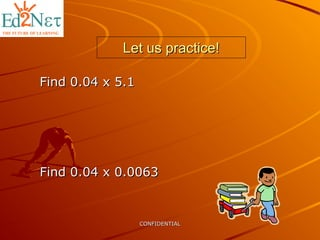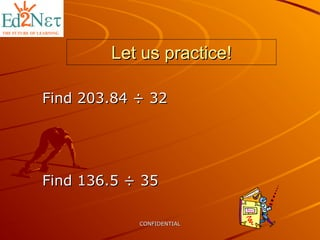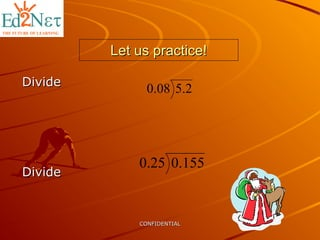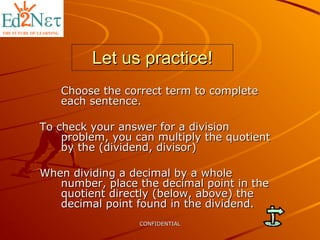1 von 29

### Chapter3.3prealg

• 1. CONFIDENTIALCONFIDENTIAL Let us warm up with some practiceLet us warm up with some practice 101055 == 2 x 14 + 2 x 6 =2 x 14 + 2 x 6 = 2 x 1 + 2 x 1 =2 x 1 + 2 x 1 = 6.8 + 6.8 + 10.2 + 10.2 =6.8 + 6.8 + 10.2 + 10.2 =
• 2. CONFIDENTIALCONFIDENTIAL Multiplying Decimals by Whole NumbersMultiplying Decimals by Whole Numbers When multiplying a decimal by a wholeWhen multiplying a decimal by a whole number, multiply as with whole numbersnumber, multiply as with whole numbers Then use estimation to place the decimalThen use estimation to place the decimal point in the product.point in the product. You can also count the number of decimalYou can also count the number of decimal placesplaces
• 3. CONFIDENTIALCONFIDENTIAL Multiplying Decimals by Whole NumbersMultiplying Decimals by Whole Numbers Method 1:Method 1: – Use estimationUse estimation Round 14.2 to 14Round 14.2 to 14 14.2 x 6 ->14x6 or 8414.2 x 6 ->14x6 or 84 14.214.2 x 6x 6 85.285.2 (Since the estimate is 84, place the(Since the estimate is 84, place the decimal point after the 5)decimal point after the 5) Example: Find 14.2 x 6Example: Find 14.2 x 6
• 4. CONFIDENTIALCONFIDENTIAL Multiplying Decimals by Whole NumbersMultiplying Decimals by Whole Numbers Example: Find 14.2 x 6Example: Find 14.2 x 6 Method 2:Method 2: – Count decimal placesCount decimal places 14.214.2 x 6x 6 85.285.2 There is one place to the right of the decimal point. Count the same number of places from right to left.
• 5. CONFIDENTIALCONFIDENTIAL Multiplying Decimals by Whole NumbersMultiplying Decimals by Whole Numbers Your turn! Find 11.4 x 8Your turn! Find 11.4 x 8
• 6. CONFIDENTIALCONFIDENTIAL Multiplying Decimals by Whole NumbersMultiplying Decimals by Whole Numbers If there are not enough decimal places in the product, youIf there are not enough decimal places in the product, you need to add zero to the left.need to add zero to the left. For example:For example: – Find 2 x 0.018Find 2 x 0.018 0.0180.018 x 2x 2 0.0360.036 Annex a zero to the left of 36 to make three decimal places. There are three decimal places.
• 7. CONFIDENTIALCONFIDENTIAL Multiplying Decimals by Whole NumbersMultiplying Decimals by Whole Numbers Your turn! Multiply 3 x 0.02Your turn! Multiply 3 x 0.02
• 8. CONFIDENTIALCONFIDENTIAL Multiplying DecimalsMultiplying Decimals When multiplying a decimal by a decimal, multiplyWhen multiplying a decimal by a decimal, multiply as with whole numbers.as with whole numbers. To place the decimal point, find the sum of theTo place the decimal point, find the sum of the number of decimal places in each factor.number of decimal places in each factor. The product has the same number of decimal places.The product has the same number of decimal places.
• 9. CONFIDENTIALCONFIDENTIAL Multiplying DecimalsMultiplying Decimals For example: Find 4.2 x 6.7For example: Find 4.2 x 6.7 4.24.2 x 6.7x 6.7 294294 252252 28.1428.14 One decimal place One decimal place Two decimal places The product is 28.14. Compared to the estimate, the product is reasonable. Estimate 4.2 x 6.7 ->4x7 or 28
• 10. CONFIDENTIALCONFIDENTIAL Multiplying DecimalsMultiplying Decimals Your turn! Multiply 0.014 x 3.7Your turn! Multiply 0.014 x 3.7
• 11. CONFIDENTIALCONFIDENTIAL Dividing Decimals by Whole NumbersDividing Decimals by Whole Numbers Dividing a decimal by a whole number is similarDividing a decimal by a whole number is similar to dividing whole numbersto dividing whole numbers
• 12. CONFIDENTIALCONFIDENTIAL Dividing Decimals by Whole NumbersDividing Decimals by Whole Numbers Divide a decimal by a 1-Digit NumberDivide a decimal by a 1-Digit Number – Find 6.8 ÷ 2. Estimate 6 ÷ 3 = 3Find 6.8 ÷ 2. Estimate 6 ÷ 3 = 3 4.3 00 8 08 6 8.62 −6.8 ÷ 2 = 3.46.8 ÷ 2 = 3.4 Compared to theCompared to the estimate, the quotient is reasonable.estimate, the quotient is reasonable.
• 13. CONFIDENTIALCONFIDENTIAL Dividing Decimals by Whole NumbersDividing Decimals by Whole Numbers Your turn! Find 3.49 ÷ 4Your turn! Find 3.49 ÷ 4
• 14. CONFIDENTIALCONFIDENTIAL Dividing Decimals by Whole NumbersDividing Decimals by Whole Numbers Divide a decimal by a 2-Digit NumberDivide a decimal by a 2-Digit Number – Find 7.49 ÷ 14. Estimate 10 ÷ 10 = 1Find 7.49 ÷ 14. Estimate 10 ÷ 10 = 1 535.0 000 070 070 42 049 70 490.714 − 7.49 ÷ 14 = 0.5357.49 ÷ 14 = 0.535 Compared toCompared to the estimate, the quotient is reasonable.the estimate, the quotient is reasonable. Place the decimal point Annex a zero and continue dividing
• 15. CONFIDENTIALCONFIDENTIAL Dividing Decimals by Whole NumbersDividing Decimals by Whole Numbers Your turn! Find 1087.9 ÷ 46Your turn! Find 1087.9 ÷ 46
• 16. CONFIDENTIALCONFIDENTIAL Dividing Decimals by Whole NumbersDividing Decimals by Whole Numbers Round a Quotient:Round a Quotient: – Usually, when you divide decimalsUsually, when you divide decimals the answer does not come outthe answer does not come out evenly.evenly. – You need to round the quotient to aYou need to round the quotient to a specified place-value position.specified place-value position. – Always divide to one more place-Always divide to one more place- value position than the place tovalue position than the place to which you are rounding.which you are rounding.
• 17. CONFIDENTIALCONFIDENTIAL Dividing Decimals by Whole NumbersDividing Decimals by Whole Numbers Round a Quotient:Round a Quotient: – Example: Seth purchased 3 video games for \$51.79,Example: Seth purchased 3 video games for \$51.79, including tax. If each game costs the same amount, whatincluding tax. If each game costs the same amount, what was the price of each game in dollars?was the price of each game in dollars? 263.17 0001 009 00010 018 0019 006 007 21 21 3 790.513 − − Place the decimal point Divide as with whole numbers Divide until you place a digit in the thousandths place. To the nearest cent, the cost in dollars is 17.26
• 18. CONFIDENTIALCONFIDENTIAL Dividing Decimals by Whole NumbersDividing Decimals by Whole Numbers Your turn!Your turn! – The table shows how much money Halley made in oneThe table shows how much money Halley made in one week for a variety of jobs. To the nearest cent, what wasweek for a variety of jobs. To the nearest cent, what was her average pay for these three jobs?her average pay for these three jobs? JobsJobs Pay in a weekPay in a week Baby-sittingBaby-sitting \$50.00\$50.00 Pet-sittingPet-sitting \$10.50\$10.50 Lawn workLawn work \$22.50\$22.50
• 19. CONFIDENTIALCONFIDENTIAL Dividing by DecimalsDividing by Decimals When dividing by decimals, changeWhen dividing by decimals, change the divisor into a whole number.the divisor into a whole number. To do this, multiply both the divisorTo do this, multiply both the divisor and the dividend by the sameand the dividend by the same power of 10.power of 10. Then divide as with whole numbers.Then divide as with whole numbers.
• 20. CONFIDENTIALCONFIDENTIAL Dividing by DecimalsDividing by Decimals Example: Find 14.19 ÷ 2.2Example: Find 14.19 ÷ 2.2 19.142.2 Multiply by 10 to make a whole number Multiply by the same number, 10 45.6 00000 0110 00110 088 0099 132 90.14122 − − Place the decimal point Divide as with whole numbers Annex a zero to continue
• 21. CONFIDENTIALCONFIDENTIAL Dividing by DecimalsDividing by Decimals Your turn!Your turn! – Divide 0.4 ÷ 0.0025Divide 0.4 ÷ 0.0025
• 22. CONFIDENTIALCONFIDENTIAL Let us take a short break!Let us take a short break!
• 23. CONFIDENTIALCONFIDENTIAL How about a jig-saw puzzle?!How about a jig-saw puzzle?! Let us see how fast you can do this…Let us see how fast you can do this… http://www.thekidzpage.com/onlinejigsawpuzzles/jigsaw-puzzles/12-piece-ji
• 24. CONFIDENTIALCONFIDENTIAL Let us practice!Let us practice! Find 12.9 x 7Find 12.9 x 7 Find 0.82 x 4Find 0.82 x 4
• 25. CONFIDENTIALCONFIDENTIAL Let us practice!Let us practice! Find 0.04 x 5.1Find 0.04 x 5.1 Find 0.04 x 0.0063Find 0.04 x 0.0063
• 26. CONFIDENTIALCONFIDENTIAL Let us practice!Let us practice! Find 203.84 ÷ 32Find 203.84 ÷ 32 Find 136.5 ÷ 35Find 136.5 ÷ 35
• 27. CONFIDENTIALCONFIDENTIAL Let us practice!Let us practice! DivideDivide DivideDivide 2.508.0 155.025.0
• 28. CONFIDENTIALCONFIDENTIAL Let us practice!Let us practice! Choose the correct term to completeChoose the correct term to complete each sentence.each sentence. To check your answer for a divisionTo check your answer for a division problem, you can multiply the quotientproblem, you can multiply the quotient by the (dividend, divisor)by the (dividend, divisor) When dividing a decimal by a wholeWhen dividing a decimal by a whole number, place the decimal point in thenumber, place the decimal point in the quotient directly (below, above) thequotient directly (below, above) the decimal point found in the dividend.decimal point found in the dividend.
• 29. CONFIDENTIALCONFIDENTIAL Good Job!Good Job!